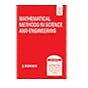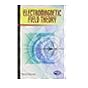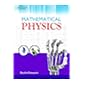Normal view

## Mathematical methods in science and engineering / by S. Selcuk Bayin

Material type:TextPublication details: New Delhi : John Wiley & sons, 2011, 2006cDescription: xxvii, 679 p. ill. ; 25 cmISBN: 9788126529681Subject(s): Mathematical physics -- TextbooksDDC classification: 530. 15
Contents:
Nature and mathematics -- Legendre equation and polynomials -- Laguerre polynomials -- Hermite polynomials -- Gegenbauer and Chebyshev polynomials -- Bessel functions -- Hypergeometric functions -- Sturm-Liouville theory -- Sturm-Liouville systems and the factorization method -- Coordinates and tensors -- Continuous groups and representations -- Complex variables and functions -- Complex integrals and series -- Fractional derivatives and integrals : differintegrals -- Infinite series -- Integral transforms -- Variational analysis -- Integral equations -- Green's functions -- Green's functions and path integrals.
Summary: An innovative treatment of mathematical methods for a multidisciplinary audience Clearly and elegantly presented, Mathematical Methods in Science and Engineering provides a coherent treatment of
Tags from this library: No tags from this library for this title.Average rating: 0.0 (0 votes)
Item type Current library Call number Status Date due Barcode Item holdsBooks Namal Library
Mathematics
530. 15 BAY-M 2011 264 (Browse shelf (Opens below)) Available 264
Total holds: 0
##### Browsing Namal Library shelves, Shelving location: Mathematics Close shelf browser (Hides shelf browser)519.77 YAD-O 2015 11534 Operations research / 519.8 GRO-F 2008 3319 Fundamentals of queueing theory 519 GLA-S 1984 919 Statistical methods in education and psychology / 530. 15 BAY-M 2011 264 Mathematical methods in science and engineering 530.1 BIE-R 1981 5971 The Racah-Wigner algebra in quantum theory / 530.141 DAW-E 2010 312 Electromagnetic field theory / 530.15 GOS-M 2012 10828 Mathematical physics/

Include bibliography references and index

Nature and mathematics -- Legendre equation and polynomials -- Laguerre polynomials -- Hermite polynomials -- Gegenbauer and Chebyshev polynomials -- Bessel functions -- Hypergeometric functions -- Sturm-Liouville theory -- Sturm-Liouville systems and the factorization method -- Coordinates and tensors -- Continuous groups and representations -- Complex variables and functions -- Complex integrals and series -- Fractional derivatives and integrals : differintegrals -- Infinite series -- Integral transforms -- Variational analysis -- Integral equations -- Green's functions -- Green's functions and path integrals.

An innovative treatment of mathematical methods for a multidisciplinary audience Clearly and elegantly presented, Mathematical Methods in Science and Engineering provides a coherent treatment of

There are no comments on this title.

to post a comment.
Share